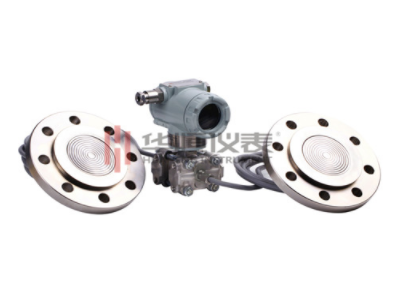##### 中国工业自动化仪表研发生产基地 精度高·可靠性高·厂价供应### 安装位置对双法兰差压式液位计参数设置的影响

双法兰液位变送器，又被叫做双法兰差压液位计，实际上也是液位计的一种。它是由测量膜片与两侧绝缘片上的电极所组成的一个电容器，可用于测量液体、气体，或者蒸汽的压力、流量、甚至密度；是一种安装于容器或者管道上的远传装置，也是一种可以防止管道中的介质直接进入变送器的压力传感器组件；它与变送器之间靠注满流体的毛细管而连接，当检测到压力货流量时，会将其转变成4～20mA DC的信号输出。1、变送器低于上下法兰口

双法兰差压式液位计安装在密闭容器低端法兰水平线以下,变送器安装在密闭容器下法兰水平线以下。

假设ρ介为介质密度=1.5，ρ0为硅油密度=0.93，H0为介质量程0-5m,H1=1m，H2=6m，求变送器的量程。

解：

量程：ΔP=ρ介×g×H0=1.5×9.8×5=73.5KPa

高压侧空液位压力：P（+）=ρ0×g×H1=0.93×9.8×1=9.114KPa

低压侧空液位压力：P（-）=ρ0×g×H2=0.93×9.8×6=54.684KPa

空液位时压力差：ΔP=P（+）-P（-）=9.114-54.684=-45.57KPa

满液位时压力差：ΔP=空液位压力差ΔP+量程值ΔP=-45.57+73.5=27.93KPa

变送器的量程为：-45.57至27.93KPa

2、变送器位于上下法兰之间

双法兰差压式液位计安装在密闭容器法兰水平线中间,变送器安装在密闭容器高低侧法兰水平线中间。

假设ρ介为介质密度=1.5，ρ0为硅油密度=0.93，H0为介质量程0-5m,H1=2m，H2=3m，求变送器的量程。

解：

量程：ΔP=ρ介×g×H0=1.5×9.8×5=73.5KPa

高压侧空液位压力：P（+）=ρ0×g×-H1=0.93×9.8×-2=-18.228KPa

低压侧空液位压力：P（-）=ρ0×g×H2=0.93×9.8×3=27.342KPa

空液位时压力差：ΔP=P（+）-P（-）=-18.228-27.342=-45.57KPa

满液位时压力差：ΔP=空液位压力差ΔP+量程值ΔP=-45.57+73.5=27.93KPa

变送器的量程为：-45.57至27.93KPa

3、变送器高于上下法兰

双法兰差压变送器安装在密闭容器高侧法兰水平线以上。

假设ρ介为介质密度=1.5，ρ0为硅油密度=0.93，H0为介质量程0-5m,H1=6m，H2=1m，求变送器的量程。

解：

量程：ΔP=ρ介×g×H0=1.5×9.8×5=73.5KPa

高压侧空液位压力：P（+）=ρ0×g×（-H1）=0.93×9.8×-6=-54.684KPa

低压侧空液位压力：P（-）=ρ0×g×（-H2）=0.93×9.8×-1=-9.114KPa

空液位时压力差：ΔP=P（+）-P（-）=-54.684-（-9.114）=-45.57KPa

满液位时压力差：ΔP=空液位压力差ΔP+量程值ΔP=-45.57+73.5=27.93KPa

变送器的量程为：-45.57至27.93KPa

结论

在仪表施工与调试当中，经常有同仁讲以上三种安装方式零点迁移量是不一样的。从上面的计算公式中可以得出，双法兰差压式液位计变送器不管安装在什么位置，其量程和零点迁移量不会随变送器安装位置改变而改变。

【返回列表】

### 【推荐阅读】↓

• 版权所有:西安华恒仪表制造有限公司 网站地图   RSS   XML   西安华恒仪表
• QQ：3036130632 咨询热线电话：029-89016663 手机：180 9235 6836
• 联系人：刘经理     E-mail：smart_cn@vip.163.com
• 工厂地址：西安市鄠邑区草堂国家级科技企业加速器工业园10号楼3C